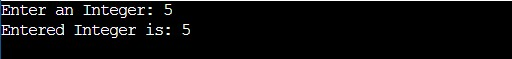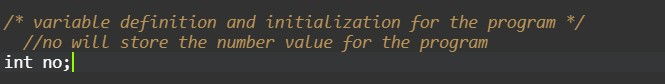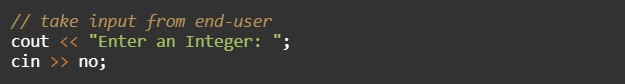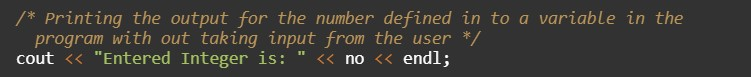# C++ Program to Print Integer

In this tutorial you will learn about the C++ Program to Print Integer and its application with practical example.

## C++ Program to Print Integer

In this tutorial, we will learn to create a C++ program that will Print Integer in C++ programming.

## Prerequisites

Before starting with this tutorial we assume that you are best aware of the following C++ programming topics:

• Operators in C++ Programming.
• Basic Input and Output function in C++ Programming.
• Basic C++ Programming.

## What is an integer?

The integer is a positive or negative whole number including zero. These are the number real numbers.

## Program to Print Integer

As we all know the integer, float, or double value in c++ programming. In c++ programming, we can take the input from the user in number format. In this program, we will take input in integer format. The second will display the integer with the help of a small program. The integer are the most useful terminology in any programing language. For number/arithmetic manipulation in c++ programming, there are many predefined functions available.

Integers are the most useful data types in any programming language. The integer values are used with operators to perform the best operations in programming.

With the help of this program, we can take input and Print Integer.

## Output:-In the above program, we have first initialized the required variable.• no = it will hold the input number value from the user.

Taking Input from the user.Printing the data in the output in the number format.In this tutorial we have learn about the C++ Program to Print Integer and its application with practical example. I hope you will like this tutorial.